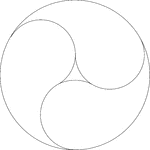### 3 Yin Yang Design Symbols In A Circle

Design made by drawing one large circle and then three circles that are internally tangent to the original…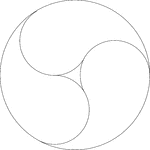### 3 Yin Yang Design Symbols In A Circle

Design made by drawing one large circle and then three circles that are internally tangent to the original…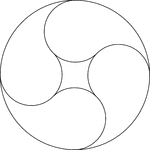### 4 Yin Yang Design Symbols In A Circle

Design made by drawing one large circle and then four circles that are internally tangent to the original…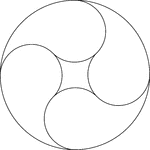### 4 Yin Yang Design Symbols In A Circle

Design made by drawing one large circle and then four circles that are internally tangent to the original…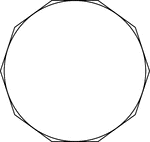### Decagon Circumscribed About A Circle

Illustration of a decagon circumscribed about a circle. This can also be described as a circle inscribed…### Decagon Inscribed In A Circle

Illustration of a decagon inscribed in a circle. This can also be described as a circle circumscribed…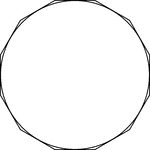### Dodecagon Circumscribed About A Circle

Illustration of a dodecagon circumscribed about a circle. This can also be described as a circle inscribed…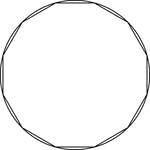### Dodecagon Inscribed In A Circle

Illustration of a dodecagon inscribed in a circle. This can also be described as a circle circumscribed…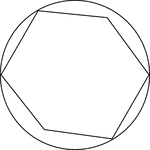### Hexagon In A Circle

Illustration of a hexagon in a circle. Four of the six vertices of the hexagon are bound by the circle…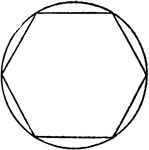### Hexagon Inscribed in Circle

Illustration of hexagon inscribed in circle. Or, circle circumscribed about hexagon.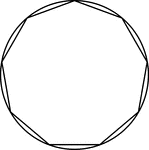### Nonagon Inscribed in a Circle

Illustration of a regular nonagon inscribed in a circle. This could also be described as a circle circumscribed…### Pentagon Inscribed in Circle

Illustration of pentagon inscribed in circle. Or, circle circumscribed about pentagon.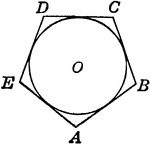Illustration of polygon circumscribed about circle. Or, circle inscribed in the polygon.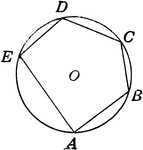### Polygon Inscribed in Circle

Illustration of polygon inscribed in circle. Or, circle circumscribed about the polygon.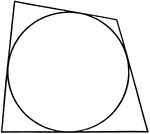Illustration of a quadrilateral circumscribed about a circle. This could also be described as a circle…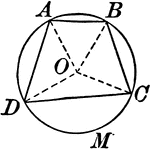Illustration showing a circle with a quadrilateral inscribed.### Quadrilateral Inscribed In A Circle

An illustration showing a quadrilateral inscribed in a circle that is tangent to a line.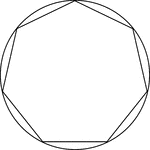### Regular Heptagon/Septagon Inscribed In A Circle

Illustration of a regular heptagon/septagon inscribed in a circle. This can also be described as a circle…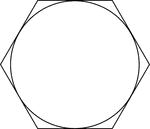### Regular Hexagon Circumscribed About A Circle

Illustration of a regular hexagon circumscribed about a circle. This can also be described as a circle…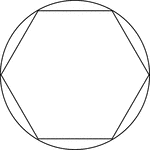### Regular Hexagon Inscribed In A Circle

Illustration of a regular hexagon inscribed in a circle. This can also be described as a circle circumscribed…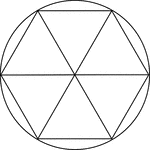### Regular Hexagon Inscribed In A Circle

Illustration of a regular hexagon inscribed in a circle. This can also be described as a circle circumscribed…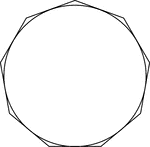### Regular Nonagon Circumscribed About A Circle

Illustration of a regular nonagon circumscribed about a circle. This can also be described as a circle…### Regular Nonagon Inscribed In A Circle

Illustration of a regular nonagon inscribed in a circle. This can also be described as a circle circumscribed…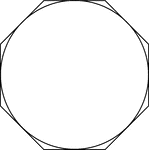### Regular Octagon Circumscribed About A Circle

Illustration of a regular octagon circumscribed about a circle. This can also be described as a circle…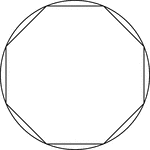### Regular Octagon Inscribed In A Circle

Illustration of a regular octagon inscribed in a circle. This can also be described as a circle circumscribed…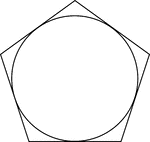### Regular Pentagon Circumscribed About A Circle

Illustration of a regular pentagon circumscribed about a circle. This can also be described as a circle…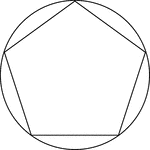### Regular Pentagon Inscribed In A Circle

Illustration of a regular pentagon inscribed in a circle. This can also be described as a circle circumscribed…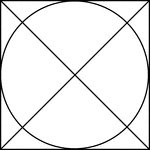### Square Circumscribed About A Circle

Illustration of a square, with diagonals drawn, circumscribed about a circle. This can also be described…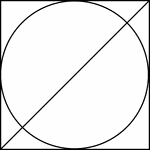### Square Circumscribed About A Circle

Illustration of a square, with 1 diagonals drawn, circumscribed about a circle. This can also be described…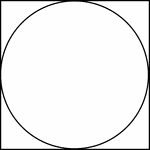### Square Circumscribed About A Circle

Illustration of a square circumscribed about a circle. This can also be described as a circle inscribed…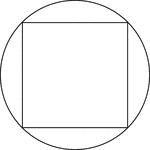### Square Inscribed In A Circle

Illustration of a square inscribed in a circle. This can also be described as a circle circumscribed…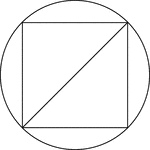### Square Inscribed In A Circle

Illustration of a square inscribed in a circle. This can also be described as a circle circumscribed…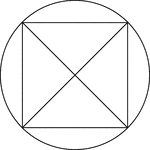### Square Inscribed In A Circle

Illustration of a square, with diagonals drawn, inscribed in a circle. This can also be described as…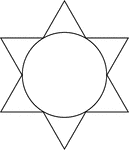### Star Circumscribed About A Circle

Illustration of a 6-point star (convex dodecagon) circumscribed about a circle. This can also be described…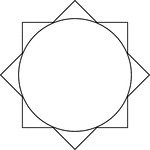### Star Circumscribed About A Circle

Illustration of an 8-point star (convex polygon) circumscribed about a circle. This can also be described…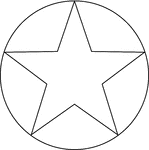### Star Inscribed In A Circle

Illustration of a 5-point star inscribed in a circle. This can also be described as a circle circumscribed…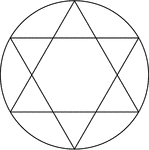### Star Inscribed In A Circle

Illustration of a 6-point star created by two equilateral triangles (often described as the Star of…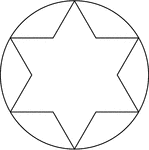### Star Inscribed In A Circle

Illustration of a 6-point star (convex dodecagon) inscribed in a circle. This can also be described…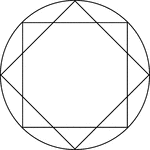### Star Inscribed In A Circle

Illustration of an 8-point star, created by two squares at 45° rotations, inscribed in a circle.…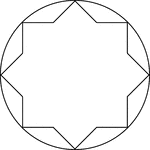### Star Inscribed In A Circle

Illustration of an 8-point star, or convex polygon, inscribed in a circle. This can also be described…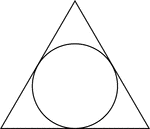### Triangle Circumscribed About A Circle

Illustration of an equilateral triangle circumscribed about a circle. This can also be described as…### Triangle Inscribed in Circle

Illustration of triangle inscribed in circle. Or, circle circumscribed about triangle.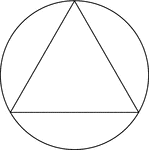### Triangle Inscribed In A Circle

Illustration of an equilateral triangle inscribed in a circle. This can also be described as a circle…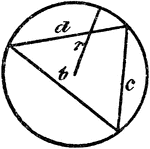### Triangle Inscribed In A Circle

An illustration showing a triangle with sides b, c, and d inscribed in a circle with radius r.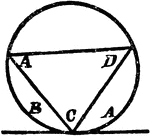### Triangle Inscribed In A Circle

An illustration showing a triangle with angles A, C, and D inscribed in a circle which is tangent to…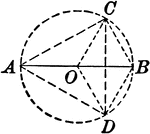### Circle With Inscribed Triangle and Radii

Illustration showing a circle with an inscribed triangle and radii.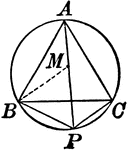### Circle With Inscribed Equilateral Triangle

Illustration showing an equilateral triangle inscribed in a circle.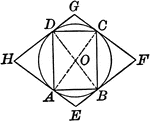### Circle With Inscribed and Circumscribed Quadrilaterals

Illustration showing a circle with an inscribed and circumscribed quadrilateral.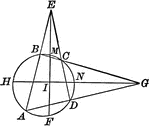### Circle With Inscribed Quadrilateral and Triangles Formed

Illustration showing a circle with an inscribed quadrilateral and triangles formed by extended chords.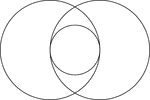### 2 Intersecting Circles

2 congruent circles whose intersection includes a tangent circle with diameter equal to the radii of…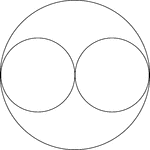### 2 Smaller Horizontally Placed Circles In A Larger Circle

A large circle containing 2 smaller congruent circles. The small circles are externally tangent to each…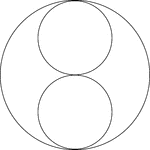### 2 Smaller Vertically Placed Circles In A Larger Circle

A large circle containing 2 smaller congruent circles. The small circles are externally tangent to each…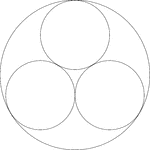### 3 Smaller Circles In A Larger Circle

A large circle containing 3 smaller congruent circles. The small circles are externally tangent to each…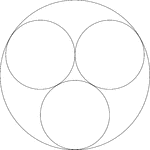### 3 Smaller Circles In A Larger Circle

A large circle containing 3 smaller congruent circles. The small circles are externally tangent to each…### 4 Smaller Circles In A Larger Circle

A large circle containing 4 smaller congruent circles. The small circles are externally tangent to each…### 4 Smaller Circles In A Larger Circle

A large circle containing 4 smaller congruent circles. The small circles are externally tangent to each…### 5 Tangent Circles

A sequence of five circles tangent to each other at a point. The radius decreases by one half in each…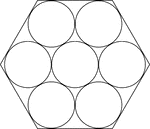### 7 Congruent Circles In A Regular Hexagon

A regular hexagon containing 7 congruent circles. The circles are externally tangent to each other and…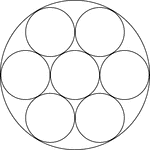### 7 Smaller Circles In A Larger

A large circle containing 7 smaller congruent circles. The small circles are externally tangent to each…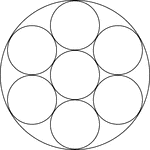### 7 Smaller Circles In A Larger Circle

A large circle containing 7 smaller congruent circles. The small circles are externally tangent to each…